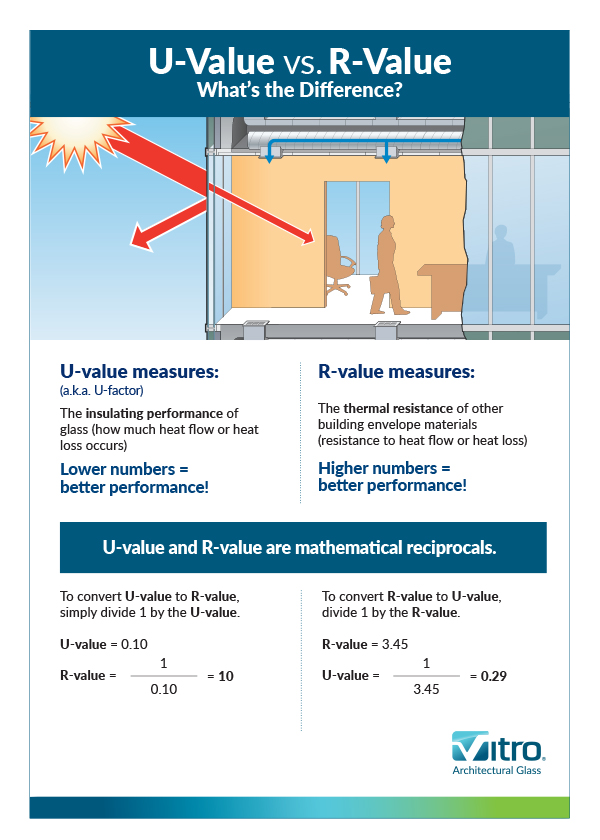# What’s the Difference Between U‑Value and R‑Value?

###### FILED IN:Low-E Glass,Infographic,Complex Applications,Understanding Performance,Science of GlassOne of the most important glass performance measures is U-value—also known as U-factor—which measures the insulating characteristics of the glass, or how much heat flow or heat loss occurs through the glass due to the difference between indoor and outdoor temperatures.

U-values can tell you how well an insulated glass unit (IGU) will hold in heated or cooled air. The lower the number, the better the insulating performance. U-values generally range from 0.1 (very little heat loss) to 1.0 (high heat loss). The U-value of a window is measured by the number of BTUs that will pass through each square foot of area per degree of temperature difference from one side of the window to the other.

### So, what is the difference between U-value and R-value? And how are they used differently?

While U-value is used to measure the performance assemblies such as IGUs, R-value is used to measure the performance of most other parts of the building envelope, such as walls, floors and roofs.

There is a relationship between U-value and R-value—they are mathematical reciprocals of each other. Thus, while lower U-values indicate better insulating performance, higher R-values indicate better thermal resistance.

To calculate R-value, divide 1 by the U-value figure. For example, a U-value of 0.10 equals an R-value of 10 (1 divided by 0.10). To calculate U-value, divide 1 by the R-value—a 3.45 R-value equals a U-value of 0.29.

### Variations and Strategies for Improving U-Value

Summer daytime U-value and winter nighttime U-value are two common derivatives of U-value.

Summer daytime U-value measures environmental conditions of a hot outside temperature and direct sunlight. A lower summer daytime U-value means the glass is better at blocking re-radiated heat, which increases the efficiency of air conditioning systems.

Winter nighttime U-value measures the environmental conditions of a cold outside temperature and no sunlight. A lower winter nighttime U-value means the glass is better at keeping heat inside, increasing the efficiency of heating systems.

Some of the ways U-values can be improved are:

• Use double- or triple-glazed IGUs
• Use a noble gas, such as argon, in the cavity between lites of glass
• Optimize the cavity size between lites of glass—a ½ " space between lites is the most widely used for both air and argon
• Specify warm-edge spacers that create an effective thermal barrier, and
• Use low-e coatings (coatings can be used on more than one surface)